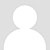《前任3》火了，背后复杂的职场人际关系却令人深思.……

《前任3：再见前任》已经突破10亿票房了，妥妥成为了2018年电影界的第一匹黑马。

01、能力强弱是成为“前任”的关键

《前任3》里有一段对话很有意思：

《前任3》虽然还有国产爱情片的坏毛病，让演员冷不丁地来一句不着调的“金句”，但总体上说，还真是个蛮接地气的电影。

“没你不还有别人吗？一个男人总会为了一个女人而去奋斗，不是吗？”02、“前任”在你心里的位置

“好像胖了点……，没我看着，就知道吃，你个猪，……以前老说我管得宽，现在你可自由了吧？03、你不在这个位置，自然就“去魅力化”了04、前任栽树，后任乘凉05、凭什么要谢别人，你要谢谢你自己

[font=Arial  ][font=Arial  ][font=Arial  ][font=Arial  ]另：法财库目前成立了多个行业微信群【银行高管群】【商业银行群】【信托群】【券商群】【理财师群】【投行群】【私募群】【基金群】【股权投资群】【期货群】【现金贷】【第三方支付】【金融高管群】【总裁群】【互联网高管群】【汽车金融群】【融资租赁群】 [font=Arial  ][font=Arial  ][font=Arial  ][font=Arial  ][font=Arial  ][font=Arial  ][font=Arial  ][font=Arial  ][font=Arial  ][font=Arial  ][font=Arial  ][font=Arial  ][font=Arial  ][font=Arial  ][font=Arial  ][font=Arial  ][font=Arial  ][font=Arial  ][font=Arial  ][font=Arial  ][font=Arial  ][font=Arial  ][font=Arial  ][font=Arial  ][font=Arial  ][font=Arial  ][font=Arial  ][font=Arial  ][font=Arial  ][font=Arial  ][font=Arial  ][font=Arial  ][font=Arial  ][font=Arial  ][font=Arial  ][font=Arial  ][font=Arial  ][font=Arial  ][font=Arial  ][font=Arial  ][font=Arial  ][font=Arial  ][font=Arial  ][font=Arial  ][font=Arial  ][font=Arial  ][font=Arial  ][font=Arial  ][font=Arial  ][font=Arial  ][font=Arial  ][font=Arial  ][font=Arial  ][font=Arial  ][font=Arial  ][font=Arial  ][font=Arial  ][font=Arial  ][font=Arial  ][font=Arial  ][font=Arial  ][font=Arial  ][font=Arial  ][font=Arial  ][font=Arial  ][font=Arial  ][font=Arial  ][font=Arial  ][font=Arial  ][font=Arial  ][font=Arial  ][font=Arial  ][font=Arial  ][font=Arial  ][font=Arial  ][font=Arial  ][font=Arial  ][font=Arial  ][font=Arial  ][font=Arial  ][font=Arial  ][font=Arial  ]
[font=微软雅黑  ]扫码加群主申请入群